# Continuous Ranked Probability Score (CRPS)¶

The Continuous Ranked Probability Score, aka CRPS, is a score to measure how a proposed distribution approximates the data, without knowledge about the true distributions of the data.

## Definition¶

CRPS is defined as1

$\operatorname{CRPS}(P, x_a) = \int_{-\infty}^\infty \lVert P(x) - H(x - x_a) \rVert_2 dx,$

where

• $$x_a$$ is the true value of $$x$$,
• P(x) is our proposed cumulative distribution for $$x$$,
• $$H(x)$$ is the Heaviside step function,
• $$\lVert \cdot \rVert_2$$ is the L2 norm.

Heaviside Step Function

$H(x) = \begin{cases} 1, &\qquad x=0\\ 0, &\qquad x\leq 0\\ \end{cases}$

## Explain it¶

The formula looks abstract on first sight, but it becomes crystal clear once we understand it.

Note that the distribution that corresponds to a Heaviside CDF is the delta function $$\delta(x-x_a)$$. What this score is calculating is the difference between our distribution and a delta function. If we have a model that minimizes CRPS, then we are looking for a distribution that is close to the delta function $$\delta(x-x_a)$$. In other words, we want our distribution to be large around $$x_a$$.

To illustrate what the integrand $$\lVert P(x) - H(x - x_a) \rVert_2$$ means, we apply some shades to the integrand of the integral in CRPS. We visualize four difference scenarios.

Scenario 1: The predicted CDF $$P(x)$$ is reaching 1 very fast.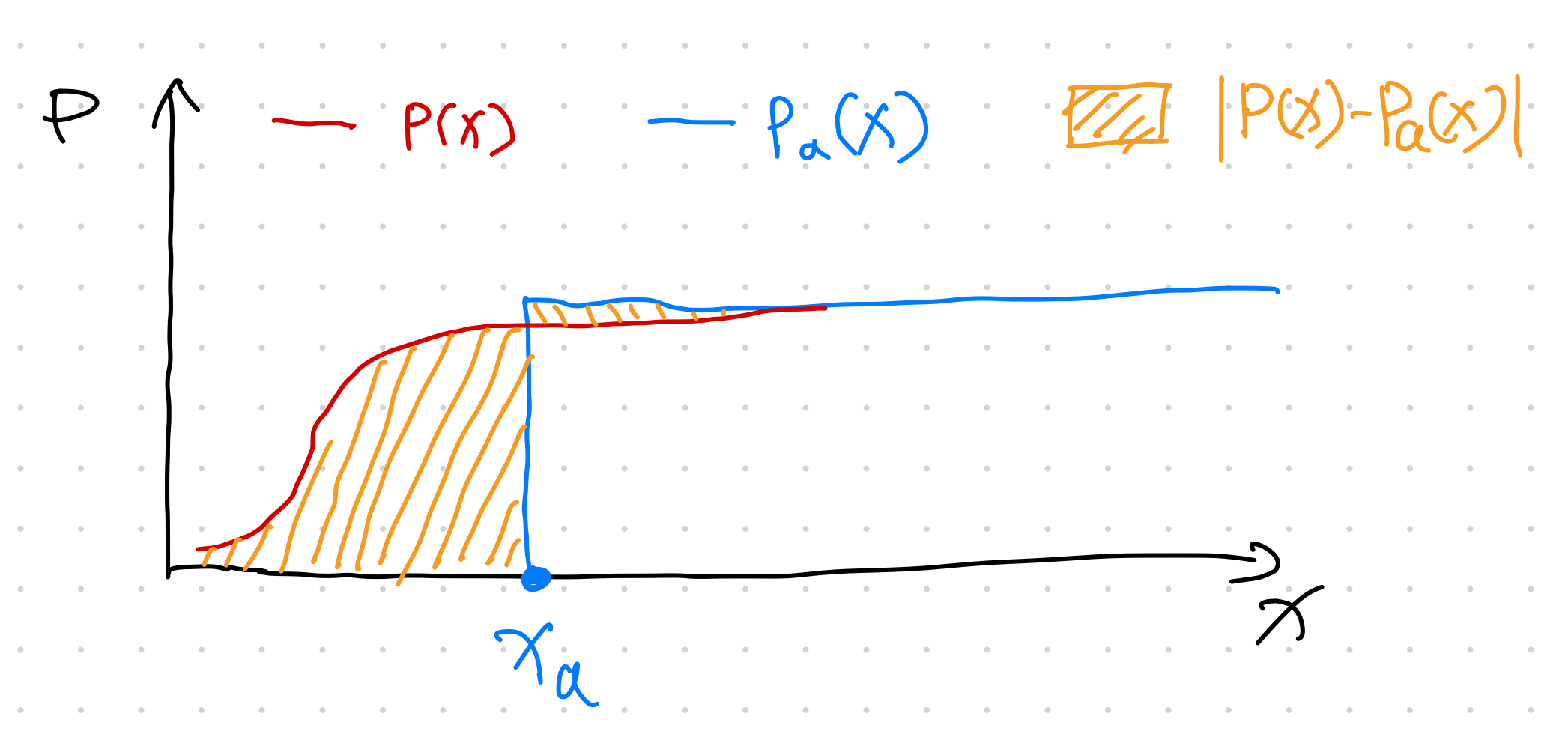Scenario 2: The predicted CDF $$P(x)$$ is reaching 1 quite late.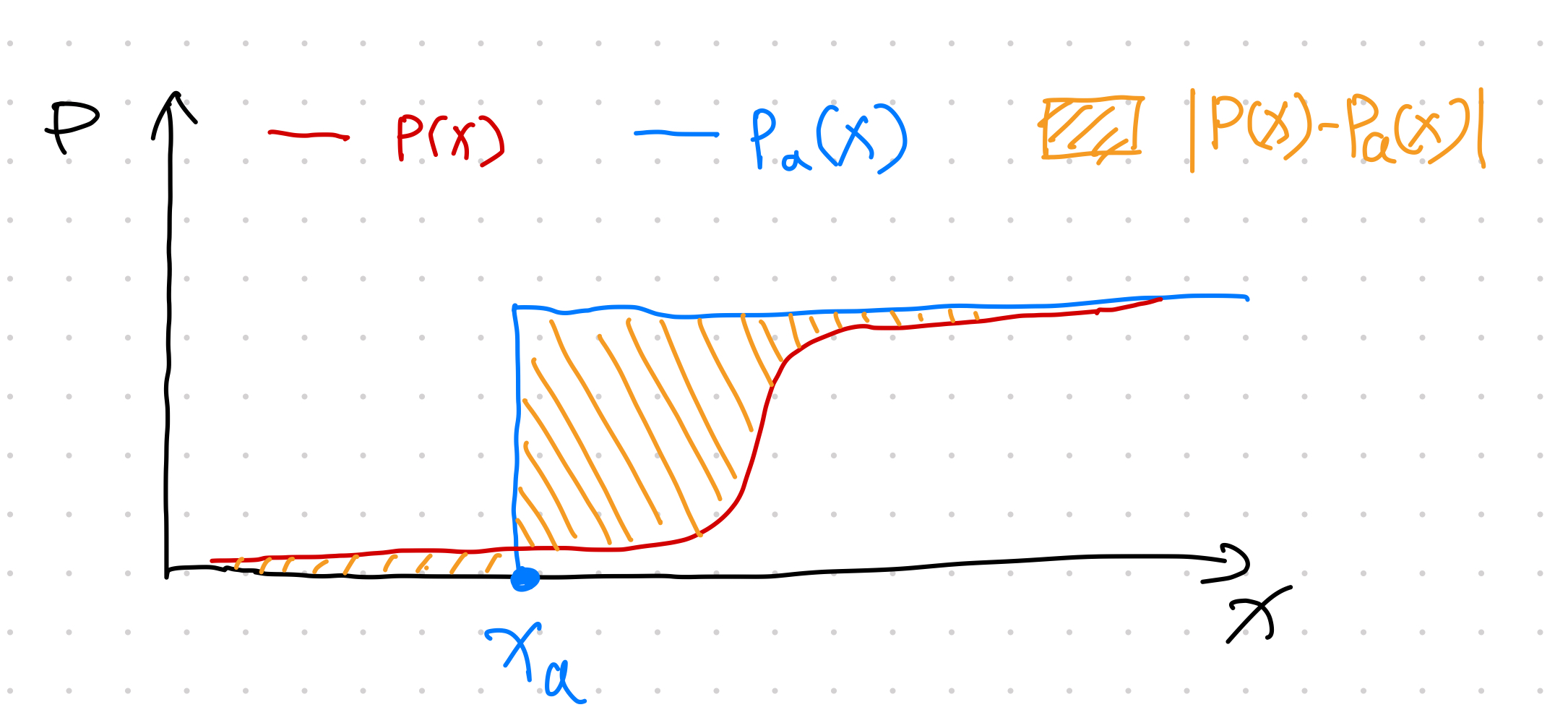Scenario 3: The predicted CDF $$P(x)$$ is reaching 1 around the Heaviside function jump.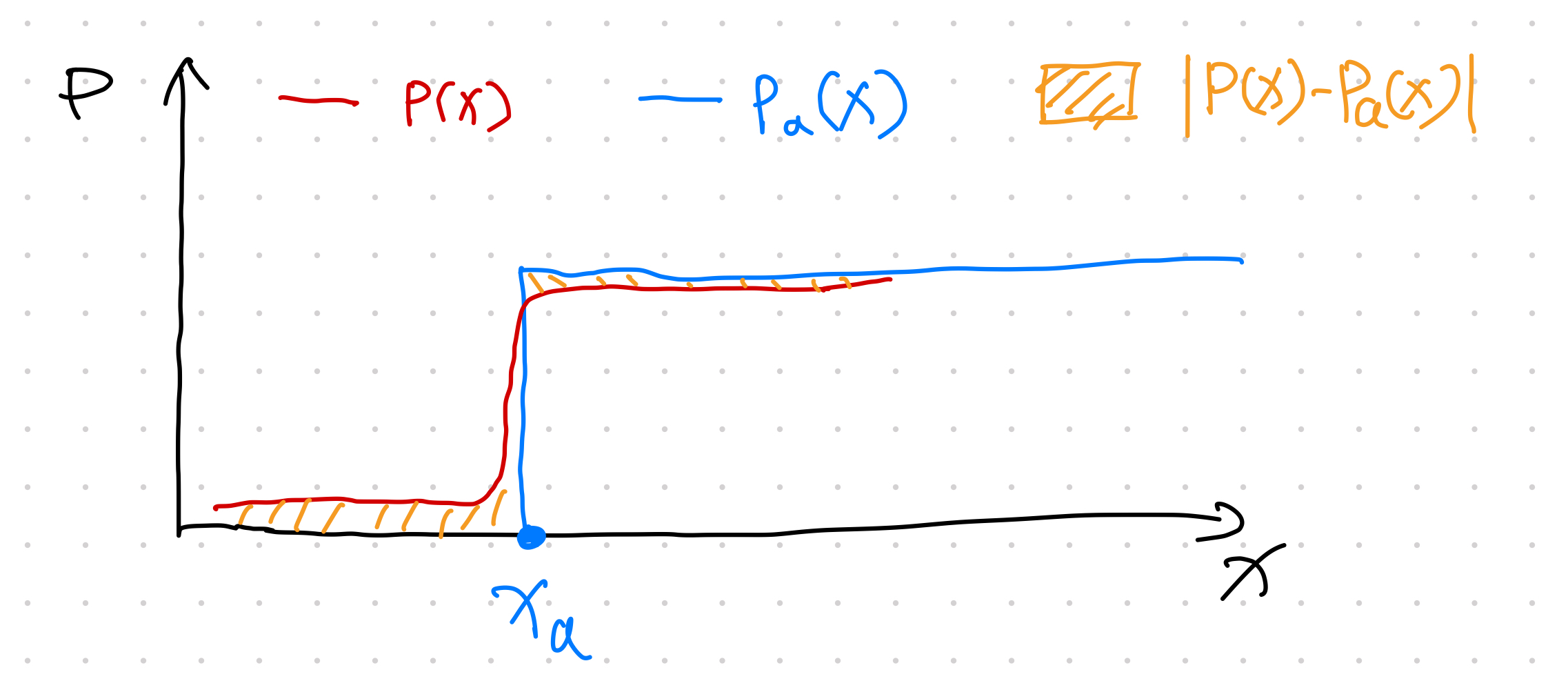Scenario 4: The predicted CDF $$P(x)$$ is steadily increasing but very dispersed.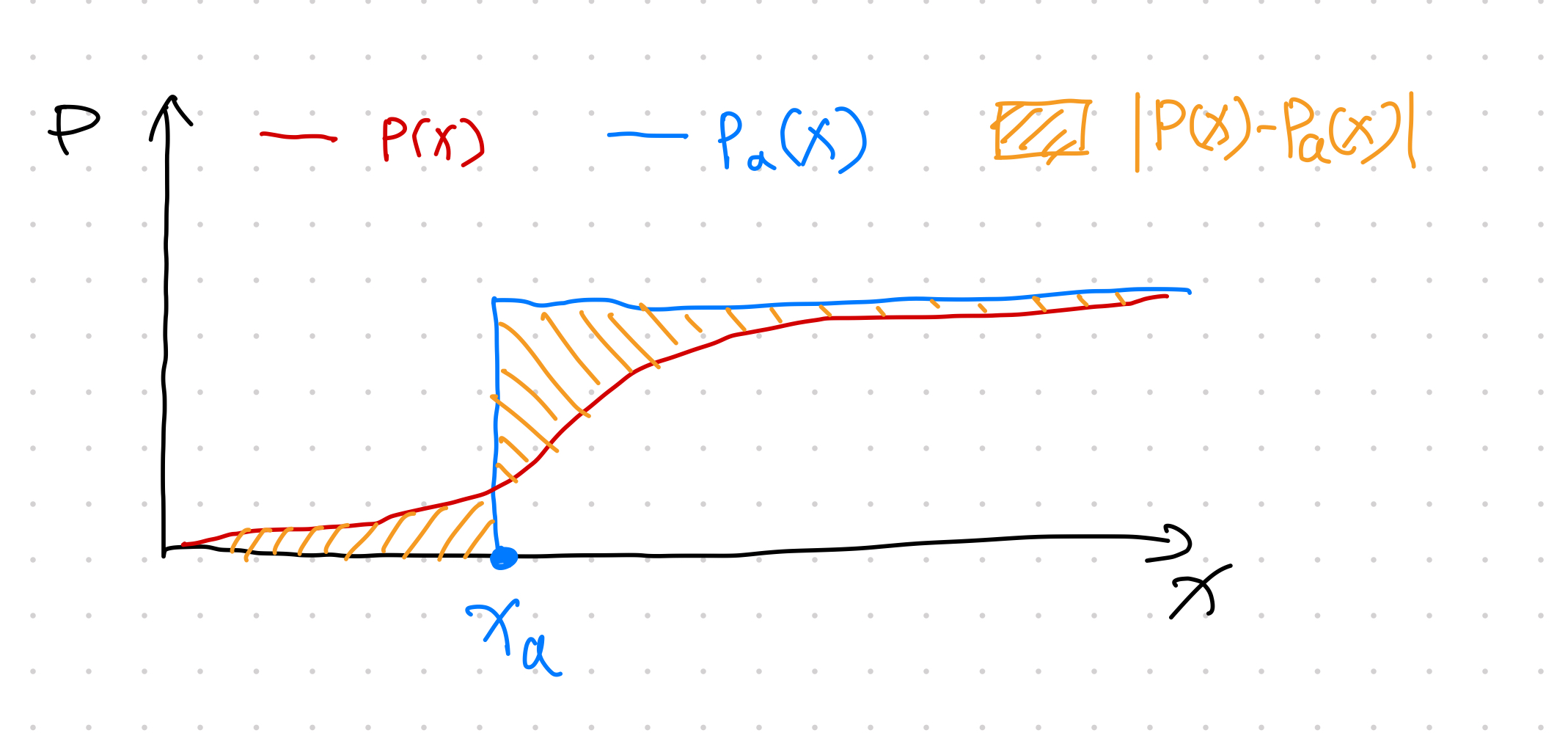With the four different scenarios visualized, intuitively, the only way to get a small CRPS score is to choose a distribution that is focused around $$x_a$$. Echoing a previous note on the delta function being the density function of the Heaviside function, we expect a small CRPS reflects a scenario of the following: the predicted distribution $$\rho(x)$$ is very focused around the observation $$x_a$$.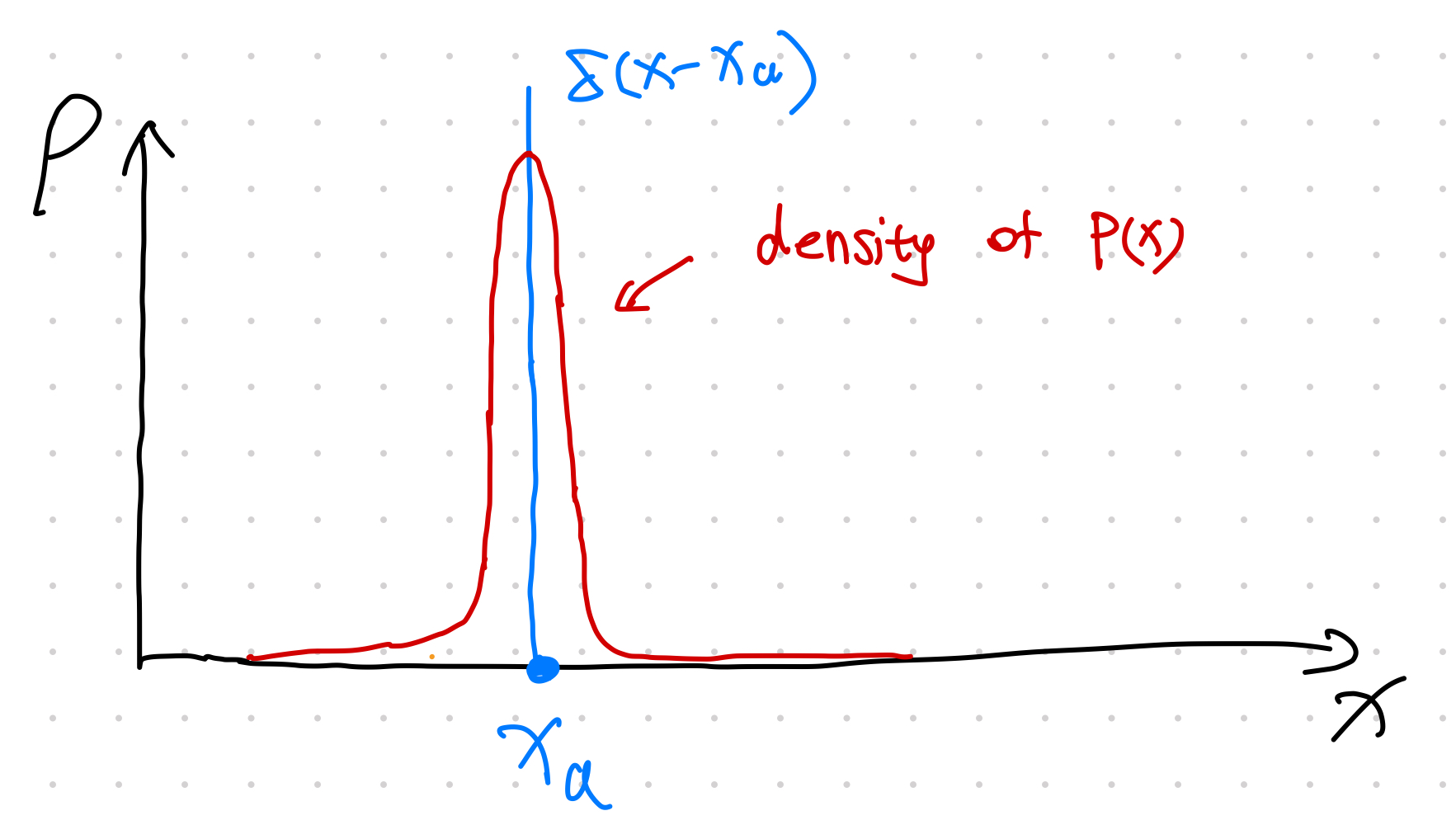## Discussions¶

Gebetsberger et al found that CRPS is more robust compared to Likelihood while producing similar results if we use a good assumption for the data distribution3.

CRPS is also very useful in time series forecasting. For example, the integrand of CRPS can be used as the loss function in model training 2.

Contributors: LM